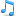Rudimentary telnet control over Pioneer AVR ReceivermanagementboyMember Posts: 81 Threads: 28 Joined: Jul 2015 Reputation: 203.07.2015, 09:19 I thought I'd share this work in progress. I am an amateur both in Lua and in KNX... so take with a huge grain of salt.  All it does is open a socket to a defined IP and Port and send a telnet command... then add an "Enter"... then wait for an answer. It closes right after that, as the Pioneer does not like more than one telnet connection at a time and I don't want to hog the port. Copy this code into a user library. I called mine user.telnet Code:```--Sends a single telnet command to a host and port --it returns one line answer function telnetsend(host, port, command)  nextline = '\r\n'  local socket = require("socket")  local tcp = assert(socket.tcp())  res, err = tcp:connect(host, port)  res, err = tcp:send(command .. nextline)  local answer = tcp:receive('*l')  tcp:close()  return answer end --Sets the volume to a numeric value --takes into account, that Pioneer increments the volume in --2 step intervals. Takes a host ip and port as input. function setvolume(host, port, volumelevel)  local currentvolume = getvolume(host, port)  if volumelevel > currentvolume then    for i = currentvolume, volumelevel -1, 2 do      telnetsend(host,port,VOLUME_UP)    end  else    for i = volumelevel, currentvolume-1, 2 do      telnetsend(host,port,VOLUME_DOWN)    end  end    currentvolume = getvolume(host, port)  return currentvolume end --Returns the current volume function getvolume(host, port)    local currentvolume = tonumber(string.sub(telnetsend(host,port,VOLUME_QUERY), 5))  return currentvolume   end --Turns reciever on off function soundonoff(host, port, onoff)  if onoff == true then    telnetsend(host,port,POWER_ON)    local status = telnetsend(host,port,POWER_QUERY)    if status == POWER_ONSTATE then      return true    else      alert("Could not turn on sound")    end      else    telnetsend(host,port,POWER_OFF)    local status = telnetsend(host,port,POWER_QUERY)    if status == POWER_OFFSTATE then      return false    else      alert("Could not turn off sound")    end  end end``` and put this code into a separate user.script. I called mine user.pioneer All it does is define a huge amount of variables with the correct telnet commands for the Pioneer avr 529 I have. Change, reduce as needed. There are most probably errors in the codes, as these where copied and pasted from around the web. Code:```   -- Main zone       POWER_ON="PO"       POWER_OFF="PF"    POWER_QUERY="?P"    POWER_ONSTATE="PWR2"    POWER_OFFSTATE="PWR0"        -- Volume    VOLUME_UP="VU"    VOLUME_DOWN="VD"    VOLUME_QUERY="?V"    VOLUME_SET="VL"        -- Mute    MUTE=    "MO"    UNMUTE=    "MF"    MUTE_QUERY="?M"    -- Source / Input channel    SOURCE_QUERY="?F"    SOURCE_UP=    "FU"    SOURCE_DOWN="FD"    SOURCE_SET=    "%02dFN"    SOURCE_DVD=    "04FN"    SOURCE_BD=    "25FN"    SOURCE_TVSAT="05FN"    SOURCE_DVR_BDR="15FN"    SOURCE_VIDEO1="10FN"    SOURCE_VIDEO2="14FN"    SOURCE_HDMI1="19FN"    SOURCE_HDMI2="20FN"    SOURCE_HDMI3="21FN"    SOURCE_HDMI4="22FN"    SOURCE_HDMI5="23FN"    SOURCE_HMG=    "26FN"    SOURCE_IPOD_USB="17FN"    SOURCE_XMRADIO="18FN"    SOURCE_CD="01FN"    SOURCE_CDR_TAPE="03FN"    SOURCE_TUNER="02FN"    SOURCE_PHONO="00FN"    SOURCE_MULTICH_IN="12FN"    SOURCE_ADAPTER_PORT="33FN"    SOURCE_HDMI_CYCL="31FN"        -- Listening mode    LISTENING_MODE="%04dSR"     LISTENING_MODE_QUERY="?L"          -- tone control     TONE_ON="TO1"     TONE_BYPASS="TO0"     TONE_QUERY="?TO"         -- bass control     BASS_INCREMENT="BI"     BASS_DECREMENT="BD"     BASS_QUERY="?BA"          -- treble control     TREBLE_INCREMENT="TI"     TREBLE_DECREMENT="TD"     TREBLE_QUERY="?TR"          -- Speaker configuration     SPEAKERS="%01dSPK"     SPEAKERS_OFF="0SPK"     SPEAKERS_A="1SPK"     SPEAKERS_B="2SPK"     SPEAKERS_A_B="3SPK"          -- HDMI outputs configuration     HDMI_OUTPUT="%01dHO"     HDMI_OUT_ALL="0HO"     HDMI_OUT_1="1HO"     HDMI_OUT_2="2HO"          -- HDMI audio configuration     HDMI_AUDIO_AMP="0HA"     HDMI_AUDIO_THROUGH="1HA"          -- PQLS setting     PQLS_OFF="0PQ"     PQLS_AUTO="1PQ"          -- Zone 2 control     ZONE2_POWER_ON=        "APO"     ZONE2_POWER_OFF="APF"     ZONE2_POWER_QUERY="?AP"     ZONE2_INPUT="%02dZS"     ZONE2_INPUT_DVD="04ZS"     ZONE2_INPUT_TV_SAT="05ZS"     ZONE2_INPUT_DVR_BDR="15ZS"     ZONE2_INPUT_VIDEO1="10ZS"     ZONE2_INPUT_VIDEO2="14ZS"     ZONE2_INPUT_HMG="26ZS"     ZONE2_INPUT_IPOD="17ZS"     ZONE2_INPUT_XMRADIO="18ZS"     ZONE2_INPUT_CD=    "01ZS"     ZONE2_INPUT_CDR_TAPE="03ZS"     ZONE2_INPUT_TUNER="02ZS"     ZONE2_INPUT_ADAPTER="33ZS"     ZONE2_INPUT_SIRIUS="27ZS"     ZONE2_INPUT_QUERY="?ZS"     ZONE2_VOLUME_UP="ZU"     ZONE2_VOLUME_DOWN="ZD"     ZONE2_VOLUME=    "%02ZV",    "ZV"     ZONE2_VOLUME_QUERY="?ZV"     ZONE2_MUTE=        "Z2MO"     ZONE2_UNMUTE=    "Z2MF"     ZONE2_MUTE_QUERY="?Z2M"          -- zone 3 control     ZONE3_POWER_ON=        "BPO"     ZONE3_POWER_OFF="BPF"     ZONE3_POWER_QUERY="?BP"     ZONE3_INPUT="%02dZT"     ZONE3_INPUT_DVD="04ZT"     ZONE3_INPUT_TV_SAT="05ZT"     ZONE3_INPUT_DVR_BDR="15ZT"     ZONE3_INPUT_VIDEO1="10ZT"     ZONE3_INPUT_VIDEO2="14ZT"     ZONE3_INPUT_HMG="26ZT"     ZONE3_INPUT_IPOD="17ZT"     ZONE3_INPUT_XMRADIO="18ZT"     ZONE3_INPUT_CD=    "01ZT"     ZONE3_INPUT_CDR_TAPE="03ZT"     ZONE3_INPUT_TUNER="02ZT"     ZONE3_INPUT_ADAPTER="33ZT"     ZONE3_INPUT_SIRIUS="27ZT"     ZONE3_INPUT_QUERY="?ZT"     ZONE3_VOLUME_UP="YU"     ZONE3_VOLUME_DOWN="YD"     ZONE3_VOLUME=    "%02YV"     ZONE3_VOLUME_QUERY="?YV"     ZONE3_MUTE=        "Z3MO"     ZONE3_UNMUTE=    "Z3MF"     ZONE3_MUTE_QUERY="?Z3M"          -- radio tuner     TUNER_FREQ_INCREMENT="TFI"     TUNER_FREQ_DECREMENT="TFD"     TUNER_FREQ_QUERY_AM="?FR"     TUNER_FREQ_QUERY_FM="?FR"     TUNER_BAND=        "TB"     TUNER_PRESET=    "%01dTP"     TUNER_CLASS=    "TC"     TUNER_PRESET_INCREMENT="TPI"     TUNER_PRESET_DECREMENT="TPD"     TUNER_PRESET_QUERY="?TP"          -- iPod control     IPOD_PLAY=            "00IP"     IPOD_PAUSE=        "01IP"     IPOD_STOP=            "02IP"     IPOD_PREVIOS=        "03IP"     IPOD_NEXT=            "04IP"     IPOD_REV=            "05IP"     IPOD_FWD=            "06IP"     IPOD_REPEAT=        "07IP"     IPOD_SHUFFLE=        "08IP"     IPOD_DISPLAY=        "09IP"     IPOD_CONTROL=        "10IP"     IPOD_CURSOR_UP=    "13IP"     IPOD_CURSOR_DOWN="14IP"     IPOD_CURSOR_LEFT="15IP"     IPOD_CURSOR_RIGHT="16IP"     IPOD_ENTER=        "17IP"     IPOD_RETURN=        "18IP"     IPOD_TOP_MENU=        "19IP"     IPOD_KEY_OFF=        "KOF"          ADAPTER_PLAY_PAUSE="20BT"     ADAPTER_PLAY="10BT"     ADAPTER_PAUSE="11BT"     ADAPTER_STOP="12BT"     ADAPTER_PREVIOUS="13BT"     ADAPTER_NEXT="14BT"     ADAPTER_REV="15BT"     ADAPTER_FWD="16BT"        -- Home Media Gateway (HMG) control    HMG_NUMKEY="%02dNW"    HMG_NUMKEY_0="00NW"    HMG_NUMKEY_1="01NW"    HMG_NUMKEY_2="02NW"    HMG_NUMKEY_3="03NW"    HMG_NUMKEY_4="04NW"    HMG_NUMKEY_5="05NW"    HMG_NUMKEY_6="06NW"    HMG_NUMKEY_7="07NW"    HMG_NUMKEY_8="08NW"    HMG_NUMKEY_9="09NW"    HMG_PLAY="10NW"    HMG_PAUSE="11NW"    HMG_PREV="12NW"    HMG_NEXT="13NW"    HMG_DISPLAY="18NW"    HMG_STOP="20NW"    HMG_UP=    "26NW"    HMG_DOWN="27NW"    HMG_RIGHT="28NW"    HMG_LEFT="29NW"    HMG_ENTER="30NW"    HMG_RETURN="31NW"    HMG_PROGRAM="32NW"    HMG_CLEAR="33NW"    HMG_REPEAT="34NW"    HMG_RANDOM="35NW"    HMG_MENU="36NW"    HMG_EDIT="37NW"    HMG_CLASS="38NW"        -- display info text    DISPLAY_INFO_QUERY="?FL"``` Lastly call the above user libraries from a KNX object like the following: Code:```require('user.telnet') require('user.pioneer') --we write the returned set volume to the status object grp.write('11/2/2', soundonoff('192.168.0.24',8102,event.getvalue()))``` The 11/2/2 Object is the status object, that gets written with the return value of soundonoff. « Next Oldest | Next Newest »